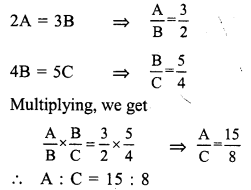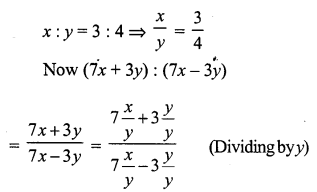# RS Aggarwal Class 7 Solutions Chapter 8 Ratio and Proportion Ex 8C

In this chapter, we provide RS Aggarwal Solutions for Class 7 Chapter 8 Ratio and Proportion Ex 8C for English medium students, Which will very helpful for every student in their exams. Students can download the latest RS Aggarwal Solutions for Class 7 Chapter 8 Ratio and Proportion Ex 8C Maths pdf, free RS Aggarwal Solutions Class 7 Chapter 8 Ratio and Proportion Ex 8C Maths book pdf download. Now you will get step by step solution to each question.

### RS Aggarwal Solutions for Class 7 Chapter 8 Ratio and Proportion Ex 8C Download PDF

Question 1.
Solution:
(d) a : b = 3 : 4, b : c = 8 : 9Question 2
Solution:
(a) A : B = 2 : 3, B : C = 4 : 5Question 3.
Solution:
(d)Question 4.
Solution:
(b) 15% of A = 20% of BQuestion 5.
Solution:
(a)Question 6.
Solution:
(b) A : B = 5 : 7, B : C = 6 : 11
LCM of 7, 6 = 42Question 7.
Solution:
(c) 2A = 3B = 4C = xQuestion 8.
Solution:
(a)
A3 = B4 = C5 = 1(suppose)
A = 3, B = 4, C = 5
A : B : C = 3 : 4 : 5

Question 9.
Solution:
(b)Question 10.
Solution:
(c)Question 11.
Solution:
(c) (3a + 5b) : (3a – 5b) = 5 : 1Question 12.
Solution:
(c) 7 : x :: 35 : 45x = 9

Question 13.
Solution:
(b) Let x to be added to each term of 3 : 5
Then 3+x5+x = 56
By cross multiplication
18 + 6x = 25 + 5x
6x – 5x = 25 – 18
x = 7

Question 14.
Solution:
(d) Ratio in two numbers = 3 : 5
Let first number = 3x
Then second number = 5x
According to the condition,
3x+105x+10 = 57
(By cross multiplication)
25x + 50 = 21x + 70
25x – 21x = 70 – 50
4x = 20
x = 5
First number = 3 x 5 = 15
and second number = 5 x 5 = 25
Sum of numbers = 15 + 25 = 40

Question 15.
Solution:
(a)
Let x be subtracted from each of the term
15–x19–x = 34
⇒ 4 (15 – x) = 3 (19 – x)
⇒ 60 – 4x = 57 – 3x
⇒ -4x + 3x = 57 – 60
⇒ -x = -3
x = 3
Required number = 3

Question 16.
Solution:
(a)
Amount = Rs. 420
and ratio = 3 : 4
Sum of ratios = 3 + 4 = 7
A’s share = 420×37 = Rs. 60 x 3 = Rs. 180

Question 17.
Solution:
(d)
Let number of boys = x, then
x : 160 : : 8 : 5
⇒ x x 5 = 160 x 8
x = 160×85 = 32 x 8 = 256
Number of total students of the school = 256 + 160 = 416

Question 18.
Solution:
(a)Question 19.
Solution:
(c)
Let x be the third proportional to 9 and 12 then
9 : 12 :: x : 12
⇒ 9 x x = 12 x 12
⇒ x = 12×129 = 1449 = 16
Third proportional = 16

Question 20.
Solution:
Mean proportional of 9 and 16 = √(9 x 16) = √144 = 12

Question 21.
Solution:
(a)
Let age of A = 3x
and age of B = 8x
6 years hence, their ages will be 3x + 6 and 8x + 6
3x+68x+6 = 49
⇒ 9 (3x + 6) = 4 (8x + 6)
⇒ 27x + 54 = 32x + 24
⇒ 32x – 27x = 54 – 24
⇒ 5x = 30
⇒ x = 6
A’s age = 3x = 3 x 6 = 18 years

All Chapter RS Aggarwal Solutions For Class 7 Maths

—————————————————————————–

All Subject NCERT Exemplar Problems Solutions For Class 7

All Subject NCERT Solutions For Class 7

*************************************************

I think you got complete solutions for this chapter. If You have any queries regarding this chapter, please comment on the below section our subject teacher will answer you. We tried our best to give complete solutions so you got good marks in your exam.

If these solutions have helped you, you can also share rsaggarwalsolutions.in to your friends.